# The Unit One in Three Dimensions Correlates Phi and Pi

From the Geometry of the Universal Mother and Father, we recall that round and curvy shapes are associated with Femininity and straight and angular shapes are associated with Masculinity.

Below, Fuxi and Nuwa, the Chinese Adam and Eve, gaze upon each other while holding tools of geometry.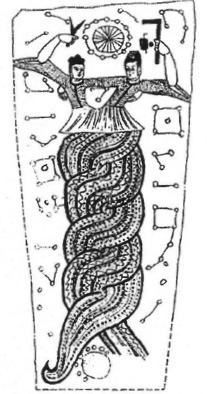Nuwa, the Female on the left, holds a compass that makes circles.  Fuxi, the Male on the right, holds a tool that makes 90° angles, four of which exist inside a square, and four of which connect the side lengths of squares.The more squares we place around a circle, the more tightly the tangential lines close in around the circle.  Theoretically, an infinite number of skewed squares would cover all the points of a perfect circle.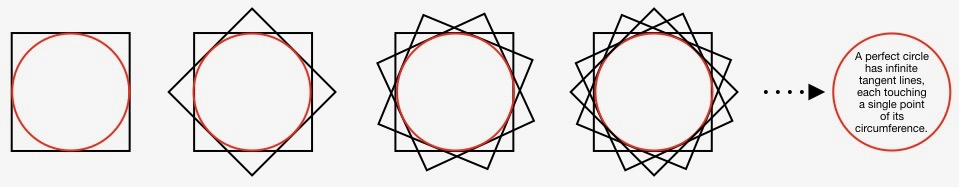Instead of thinking that numerous squares are situated around a circle, as seen above, let us instead pretend that the four corners of a single square whirl around a central axis like fan blades.  Imagine this axis is like a wheel axle; it runs perpendicularly through the black dot seen in the image below.The smooth sides of the square trace out the red in-circle and the pointy corners of the square trace out the orange ex-circle.

#### Calculating Measurements

The side lengths of the square measure the same as the diameter of circle inscribed within it.  To this length, we assign 1 linear unit.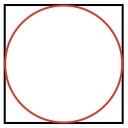The diagonal of the square measures the same as the diameter of the ex-circle traced out by the corners of the square.The diameter dissects the square into two right angle triangles.  Given that sides (AC) and (CB) each measure 1 linear unit, what is the length of the diagonal AB, which is also the diameter of the orange ex-circle?

To answer that question we employ the Pythagorean Theorem: a² + b² = c²

Pythagoras tells us that on a right angle triangle, the sum of squares formed on each of the two sides, ‘a’ and ‘b’ equals the number of squares formed on the hypotenuse, ‘c’.  Below, we see the geometric evidence that 4² + 3²  = 5² (16 + 9 = 25).Given the length of one linear unit each for the two sides AC and BC, we can calculate the length of the hypotenuse AB of the triangle ABC, shown again just below.First, the side AC, which equals 1 linear unit, can be renamed as line segment ‘a’.  The side CB, which also equals 1 linear unit, becomes line segment ‘b’.  The hypotenuse AB becomes line segment c.

a² = 1 x 1, or 1 sq. unit.  b² = 1 x 1, or 1 sq. unit.  Add the squared values together:  1 + 1 = 2 square units.  The square of the hypotenuse ‘c’ then equals 2 sq. units.  Deduce the length of hypotenuse ‘c’ by taking the square root of those 2 sq. units.  The hypotenuse AB of triangle ABC has a length of √2.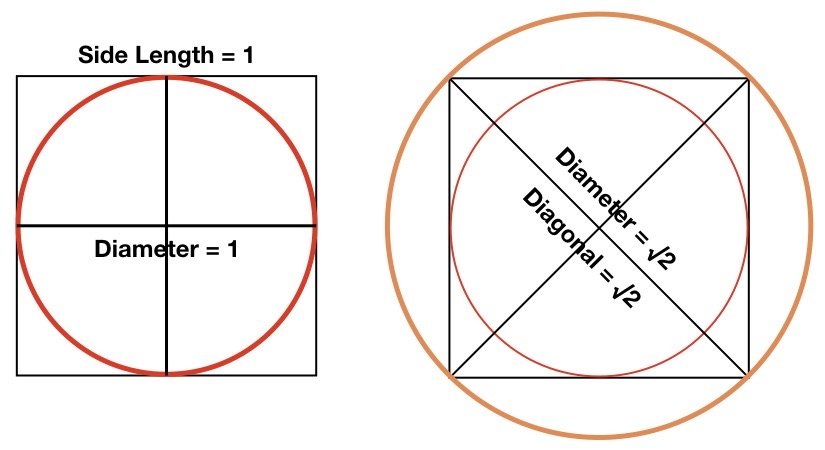So far we know that:

• the diameter of the inner/red circle is 1, or √1.
• the diameter of the outer/orange circle is √2, and
• The square touches and communicates between both the inner and outer circles.

When a square is situated between two concentric circles, touching them both, as seen below,  the total area contained within the grayed out rim is precisely equivalent to the area of the central white disc.This is true only of the square; no other polygon produces this particular equivalency.

As seen below, a polygon with three equal sides (triangle to the left of the square) has a central disc containing less area than in its rim.  A polygon with five equal sides (pentagon to the right of the square), or any polygon with more than five equal sides, has a central disc with an area greater than the area in its rim.  Only a polygon with four equal sides (a square) produces equal areas in both the outer rim and inner disc.The round (feminine) lines of the circles and the straight (masculine) lines of the square may be alternated in this fashion, ad infinitum.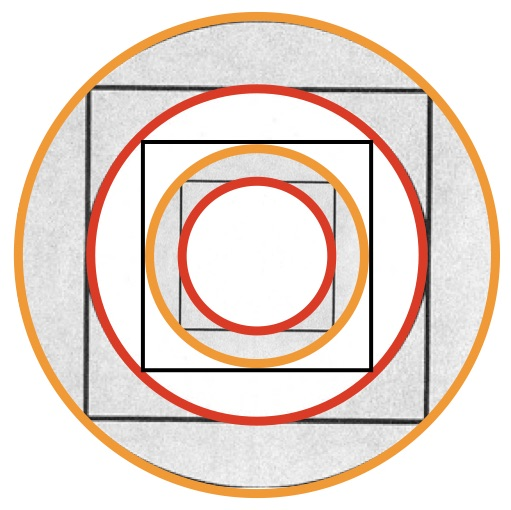Fantastic insights have been hidden in plain sight; in fact, this relationship of equality has been hidden right under our own noses (and mouths), in dinner plates of all things!

A few of you may be asking, “How is this so?  Show me the math!”  If you are not one of those people, you can skip down to where the bolded formulas end.The formula to calculate the area within a circle is pi (~3.14159) times the square of the circle’s radius.

We will take the easy one first.  The red circle has a radius of .5 because its diameter is 1.  .5² is .25.

3.14159 x .25 = .7853875

The orange circle has a radius of 1/2 the √2 (~1.414213562 / 2 = .707106781).  That radius squared is .707106781² = .5

3.14159 x .5 = 1.570795

We divide the total area inside the orange circle by the area inside the red circle to find their relationship

1.570795 / .7853875 = 2

That makes sense because .5 is twice .25.

Subtract the area inside the red circle from the total area within the orange circle.

1.570795 – .7853875 = .7853875

This confirms that the area of both the rim and the disc are the same, equal to .7853875 of one square unit.

Was Fuxi and Nuwa telling us with their right angle and compass that they are equal partners in creation and that their dynamic relationship operates continuously across all dimensions from the infinitesimal to the infinite?  I think so.

In this video we see an ancient Mongol cloth that shows the proportions of equality were known long ago.

#### Inside and Outside Spaces

Below, you can actually see an illuminated magnetic field.  On the outside of the ring magnet (dark ring) we see the effect of centrifugal force (outwardly Pushing), and on the inside of the ring we see the effect of centripetal force (inwardly Pulling).In this video from YouTube you are able to actually see a 3D holographic image of the toroidal field looping around, combining centripetal and centrifugal forces in constructive and destructive interference.

The graphic below simply shows the direction of the opposing forces with a neutral white annulus between the outer red circle and the inner teal circle.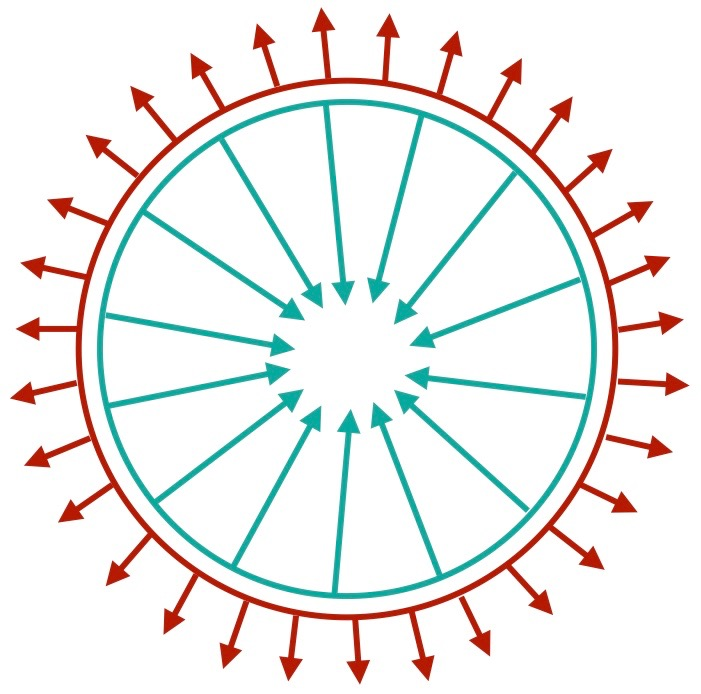Now it’s time to pull out our handy Pragmatic Schematic (PS).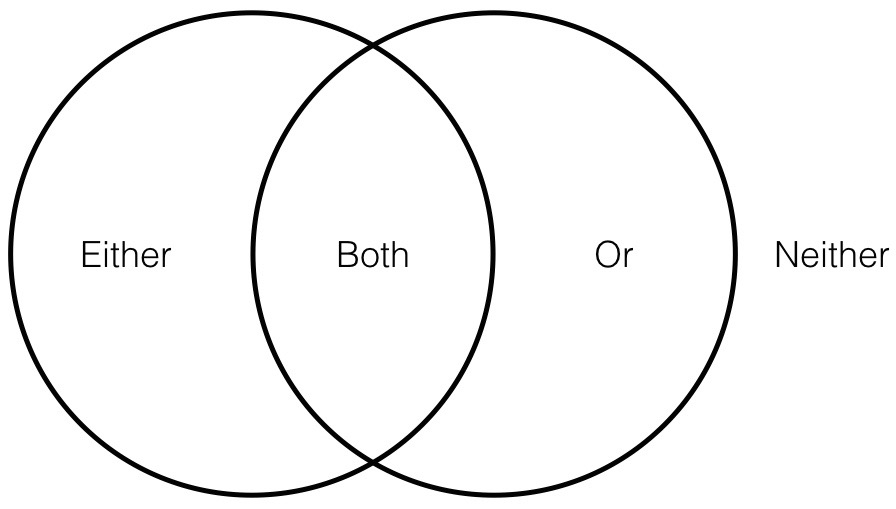The outer crescents represent two opposites.  The central area of overlap represents what the two extremes have in common, their middle ground.  Beyond the PS is its environment, its Observer, or that by which it is sustained.

Understanding that centripetal (INward Pull) and centrifugal (OUTward Push) are opposite forces, we can think of them in the opposite (Either * Or) crescents.  The boundary between the opposites (depicted as an annulus in the simple graphic and the toroidal interference patterns in the photograph above) go in central mandorla, or the area of overlap (Both), indicating the Transition between forces.  In this example, we, as observers, are in the transcendent space (Neither).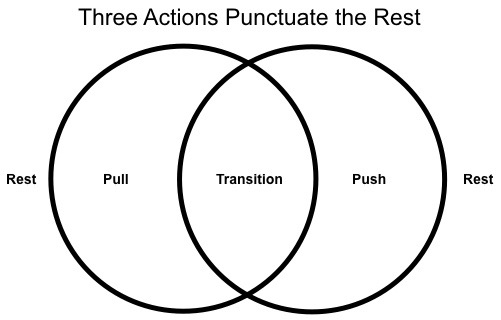Bubbles are all boundary.  There is no difference between the air inside and the air outside the bubble, other than the fact that centripetal pressure is exerted on the inside space by the boundary containing it, and centrifugal pressure is exerted on the outside space by the boundary defending itself.  These opposing forces are achieved by the surface tension, or the viscosity of the soapy substance from which the bubble is made.Here is a fun aside: Brilliance of Bubbles.

#### Transformation: From 2D to 3D

Quadernity has taught us that OUTformation is fractal, or self-similar on all scales.  Notice in the two images below, the tendency of clumping connected by linear filaments.  The neurons are too small to be seen without a powerful microscope.  Super galactic structures are too vast to be seen without powerful computers to simulate them.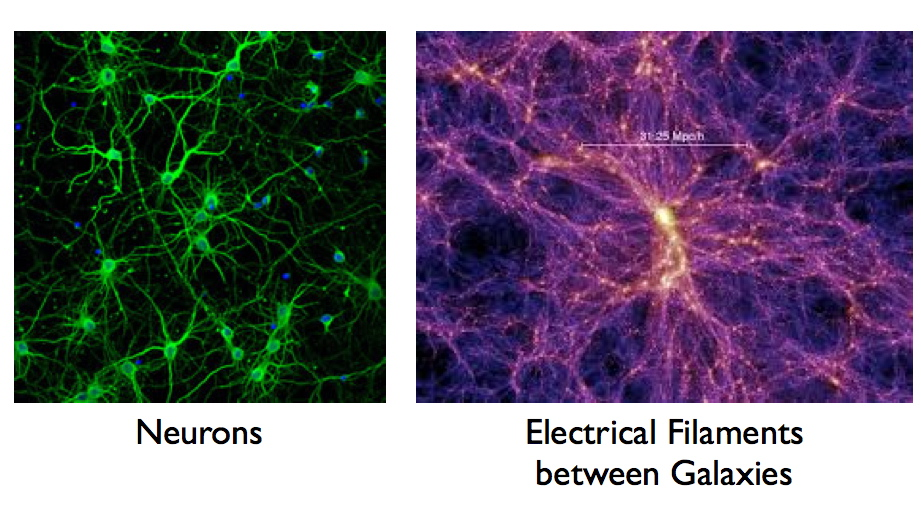Now check out ordinary bubbles that we can see with our own eyes.  Notice how the smaller bubbles clump together and the edges of the larger bubbles look similar to the linear filaments.What looks like lines, in the photo above, are actually the edges of surfaces.

Here is what I mean: if we look directly down upon a cube, we would see only its top surface, missing the third dimension beneath it.  The square surface we would see has four corners that connect the sides at 90º angles.Change the angle of our perspective and we see the cube differently.  What had appeared before as the four sides are now understood to be corners as well, connecting adjacent surfaces at 90º angles.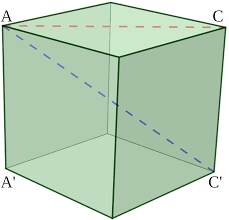So let us compare and contrast our two-dimensional Pragmatic Schematic, a tool of logic, to a three-dimensional example of two spheres sharing a boundary in common.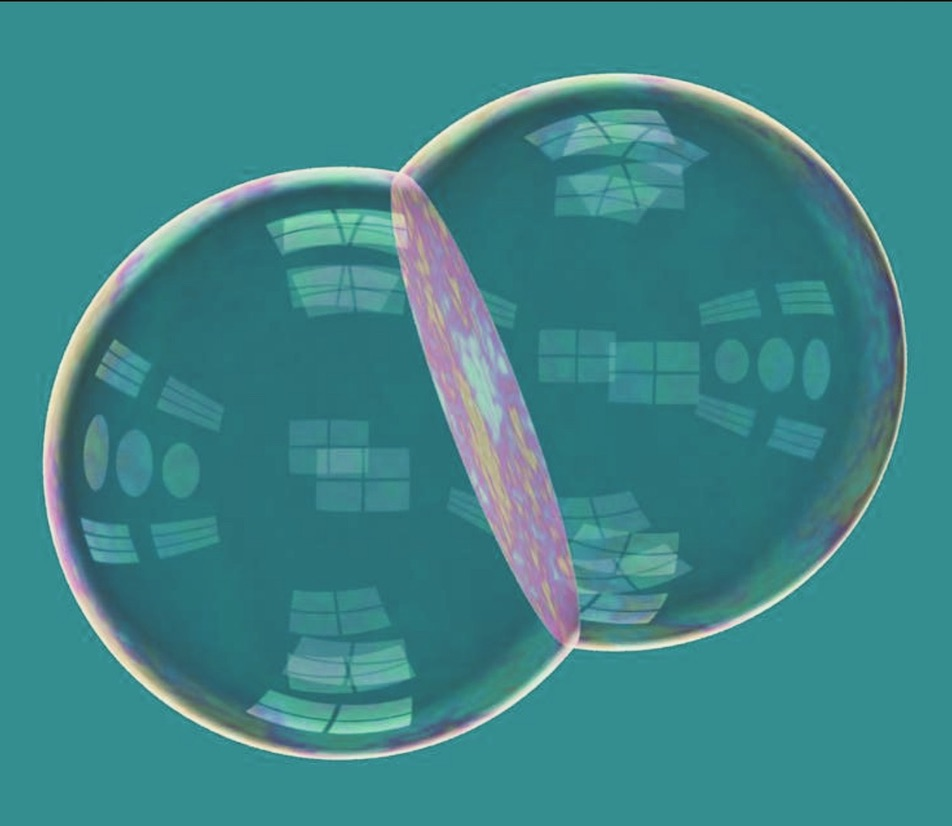Whereas the area of overlap in the Pragmatic Schematic, a tool of logic, is an almond-shaped space stretched open to allow for labeling whatever the two opposite crescents have in common, actual three-dimensional bubbles share a two-dimensional boundary (see the graphic just above).  Keep in mind that if instead of seeing the bubbles from an angle, we looked at them from a straight-on view, the pink-highlighted, disc-like surface would appear as a straight line, just as we saw in the previous photo of real bubbles.

#### Cube Fits into Spheres

We are about to discover what our cube, with side lengths of 1 linear unit, has in common with the PS, whose overlapping circles have radii of 1 linear unit.

The diagonal AC on the two-dimensional surface of the cube below has been established as √2.  We want to know what the diagonal measures from upper corner A to the opposite lower corner C’, as it passes through three dimensions instead of two.Two black diagonal lines have been added to the cube below.  They emphasize that the blue dashed diagonal AC’ is simply the diagonal through a rectangular plane.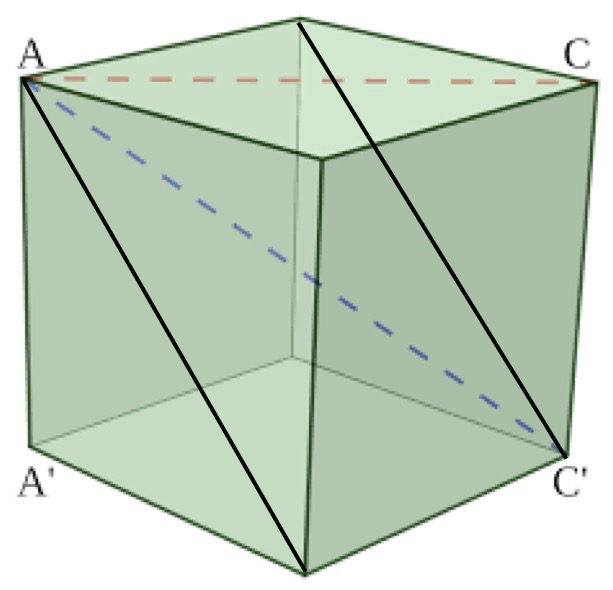Because the rectangle has right angles, we can again use the Pythagorean formula.  We know that the side lengths of the cube are 1 linear unit.  We also know that the diagonal though any of the six faces of the cube measures √2.  1² = 1.  √2² = 2.  1 + 2 = 3 square units.  AC’ is therefore √3.

Brownie points to all of you who remember that the vertical measure of the mandorla, from point to point, is also √3!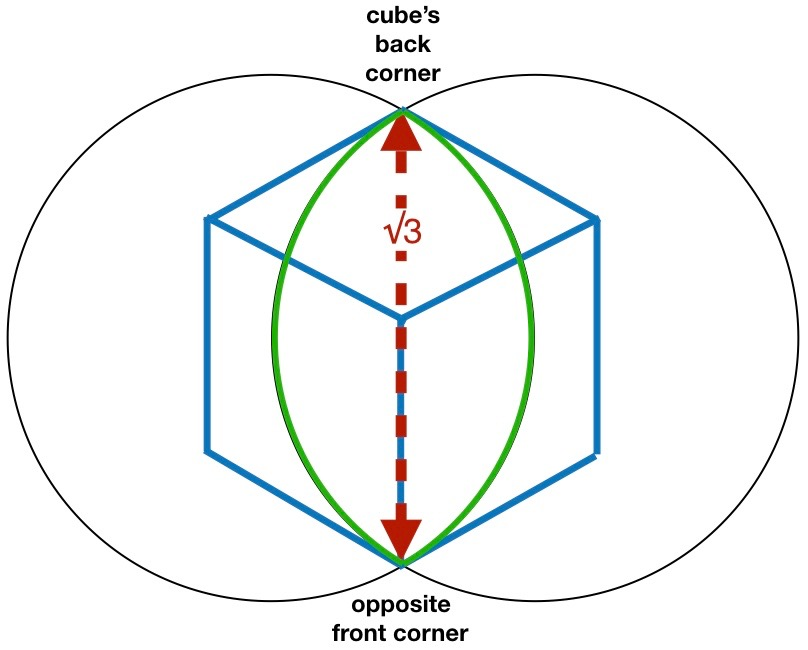When we continuously rotated a square with a diagonal of √2 around a central axis, we got a circle with a diameter of √2.  Both the square and the circle are 2D objects.  Their shared plane is perpendicular to the axis around which the rotation occurs.

Just as spinning a 2D square around its center-point of its diagonal produces a circle with a diameter of √2, rotating a 3D cube around the center-point of its diagonal would trace out a circle measuring √3.

The square’s diagonal is confined to two-dimensions, so rotating a square is likewise confined to those two dimensions.  Because a cube is three dimensional, it can be swiveled about its geometric center, in every direction permitted within 3D space.  Doing so will produce a sphere, rather than a circle.  The 3D sphere will also have a diameter equal √3.A 3D cube (Male) with the diagonal of √3, which is ~1.732 linear units, sits within its 3D sphere (Female).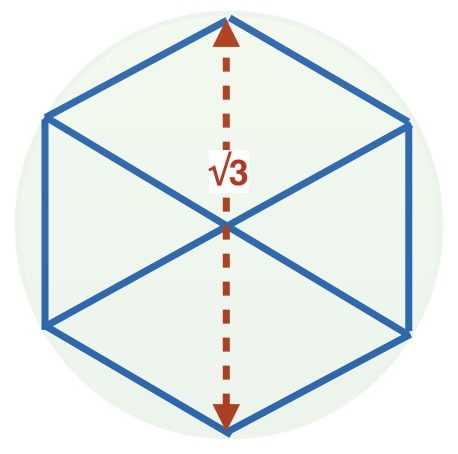Imagine the 2D PS above is two 3D bubbles sharing a disc-like surface.  As seen straight-on, the red-dashed arrow would indicate the diameter of that disc, as seen edge-on.

####Square Roots as Axes of Spin

• The 3D cube has an axis √3 times the length of its edges.
• The 2D square has an axis √2 times the length of its edges.

Following this pattern, a 1D line’s diagonal axis should be √1 times as long as its length.

Because the diagonal would be constrained necessarily to only the 1D space occupied by the line, the diagonal and the line coincide.  The line’s axis and its length are thus identical: √1 = 1.

The axis that the line spins upon is identical to itself.  It has what is called intrinsic spin, a term associated with fermions (matter particles have 1/2 integer spins) and bosons (photons and force carriers have integer spins: 0,1,2 etc.).

I suggest we think of intrinsic spin in a revolutionary way (pun intended!).

Electrons are described as zero-dimensional point particles.  Regardless, physicists believe that electrons produce real-world effects, such as magnetic moments.

This quote, from the intrinsic spin article linked above, states that only when enough of the electrons “agree” and line up with each other is a magnet formed:

Electrons do each have a magnetic field (called the “magnetic moment” for some damn-fool reason) [their words not mine], as do protons and neutrons.  If enough of them “agree” and line up with each other you get a ferromagnetic material, or as most people call them: “regular magnets”.

To produce a magnetic moment, physicists declare that the electrons must be spinning. The problem with point-particles spinning is that point-particles have neither a geometric center, nor a radius of anything else to spin around it.  It is meaningless to say that 0D point-particles spin.

In order for the physicists to differentiate the spin purported to be possessed by subatomic particles, from the normal, rotate-around-an-axis-kind-of-spin, they came up with the term “intrinsic spin“.

The giveaway in the quote above is that magnets require “enough” electrons to “line up”.  It is possible that the physicists are thinking in a reductionist way, as they often do.  They may be assuming that the magnet gets its magnetic field from a build up of “enough” electrons.  If they believe the electrons are collectively the source of the magnet’s field, then they might assume that each electron has a magnetic moment, which would then require the impossible spin.  Even though they realize a point particle itself cannot really spin (as in having angular momentum), they just made up a new term “intrinsic* spin” and kept on truckin’.

*The word intrinsic is defined as: belonging to a thing by its very nature.  This definition is accurate to the 1D line, for reasons I have described above; however, this definition does not apply to 0D particles that have no axis at all around which they could possibly rotate.

If ferro-magnetic material is made up of “agreeable” electrons, all “lined up”, then it seems more likely to me that it could be the line that has intrinsic* spin, and that when the electrons fall in line the magnetic field appears.  Spin may not be a property of point particles, but a property of the line into which they are attracted, and through which their charges get shuttled.

#### Spatial Dimensions

Throughout this blog book I use the word ‘dimension‘ differently than most.

To go conceptually from a one-dimensional line to a two-dimensional plane, we take the length of the line, say 2 linear units, from an exponent of one to an exponent of two.  If the original line measured 2 linear units, then 2¹ means we take the unit (always one) and multiply it by two one time.  1 x 2 = 2 linear units.

2² means we take the unit (1) and multiply it by two, two times.  1 x 2 x 2 = 4  Now we say we have square units.  We went from linear to square units by changing the direction of the second 2 by 90°.

2³ means we take the unit (1) and multiply it by two, three times. 1 x 2 x 2 x 2 = 8 cubic units. Now we say we have cubic units.  We went from square units to cubic units by changing the direction of the third 2 again by another 90º.

It is quite difficult to visualize what would happen next–if we were to try to change directions again by 90º.  That does not mean that 2^4 is not a real number; 1 x 2 x 2 x 2 x 2 = 16.  2^4 does not generally evoke a geometric continuum.  The three ‘dimensions’ as we commonly use the word, are rather self-limiting.

Lines, surfaces, and even a six-sided cube are all mathematical objects, not real things.

Check out the aside: Mathematical Objects.

Here, I would refer you also to a previous chapter for review: Quadernity’s Nature-Based Number Line.  This number line, rather than being centered on zero, with half of its numbers as negative values, centers itself on a number (1.618º) with a zero exponent (giving it the value of 1).  Virtually anything can be represented by the number 1.  The number line proceeds to the left with with negative exponents giving fractions approaching zero.  The number line proceeds to the right with positive exponents making compound numbers that approach the infinite.  The exponents refer to internal division (fractional Parts to the left of central unit) and external multiplication (Participation in greater wholes, compound numbers, to the right of the central unit).

According to Quadernity, in total, and according to the Nature-Based Number Line, the Male, regardless of scale or exponential dimension, remains always in communication with the Female.

#### Symbolizing Female and Male Geometrically

We have throughout this blog-book used the Pragmatic Schematic as a model anytime we are comparing two opposites that share something in common.  We could use the PS to illustrate both space and time.  Space would be represented in the PS with inside and outside spaces in the two outer crescents and their boundary which divides them in the mandorla.  Time would be represented in the PS with ‘before’ and ‘after’ in the outer crescents and ‘during’ in the mandorla.

Following the geometry suggested by Fuxi and Nuwa, the three spatial dimensions (inside, boundary, and outside) are instead represented below by the circle or sphere (Female), and the three dimensions of time (before, during, and after) are instead represented by a time-line (Male).Who observes 3D (volumetric) entities?  Conscious entities do!  We do!  Consciousness transcends the hierarchy of the three lesser spatial dimensions.

Something that registers in Consciousness is ordered in time.  To be conscious of anything, it must be in contrast to something else.  Either there are two different objects, or experiences, or moments in time (past and present), or we differentiate some content from its context.  In all cases, both things compared must be put side-by-side in the consciousness of the Observer doing the comparison.

We will now represent two contiguous compared/contrasted elements as 3D bubbles (Female) that share a common surface between them.One cube with side lengths of 1 linear unit would fit snugly between the two spheres as its √3 diagonal fits precisely from point to point within the mandorla; however, putting such a cube inside each of the two spheres, which have diameters of 2 (radius of 1), would just rattle around loosely inside.For minimal conscious experience, both of the elements (spheres) must be compared and contrasted; for that, reliable contact would be required.

We have aligned the 1D measurements of the cube (side length of 1 linear unit) with the sphere (radius of 1 linear unit).

However, to be meaningful, the spherical volumes (representing the apprehended) and the cubic volumes (representing two contiguous instantiations) need to be firmly conjoined.

When one linear unit was spun around 180° in opposite directions from each of its poles, it created two overlapping circles (our 2D Pragmatic Schematic).  Each of those two circles (in the PS) has a radius of one linear unit.  Therefore, the spheres have a diameter of 2, or twice the radius of 1.

When we brought the 3D cube into play with the 2D PS, they correlated via √3.  Both the diagonal of the cube as well as the length, point to point, of the mandorla measure √3.  However, the cube extended into a dimension not contained by the PS.

We want to correlate the 3D spheres with the 3D cubes that fit inside them, and do so in such a way that establishes unity in all three dimensions.

We want the unit of 1 to be maintained on the 1D, 2D, and 3D levels.  And because we are including transcendent Consciousness, we anticipate the necessity of a fourth dimension.

The spheres have radii of 1, so their diameters are 2 linear units.  2 is equivalent to √4.  Therefore, each sphere’s diameter can be written either as 2 or as √4.  From the front upper right corner to the back lower left corner, the measurement is √4, as seen in the image below.

Can we somehow stretch the cube so that its diagonal grows from √3 to √4, making it fit snugly within the spheres?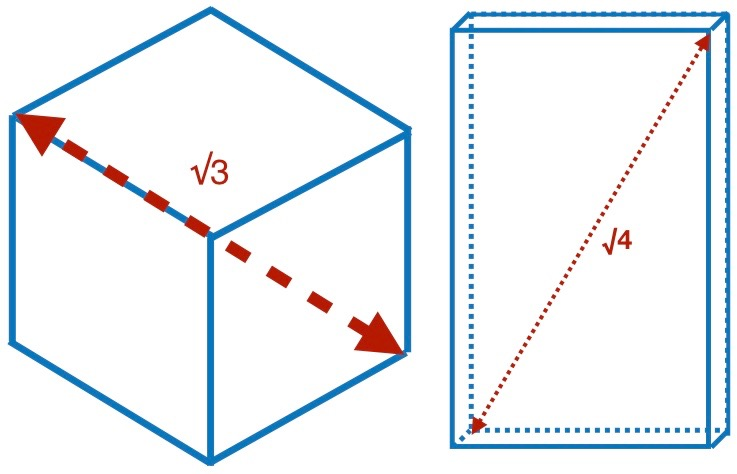Being so stretched, can the volume of one cubic unit be maintained?

Can we preserve a surface area of 1 square unit for each prism, so that their faces align contiguously in the center of the mandorla, a.k.a. Vesica Piscis?

Can the width and length of this one square unit surface fit perfectly within the √3 diameter of the shared disc-like surface already existing between the two spheres?

If all of this is achievable, our geometric symbolization will show us a cooperative union, as well as an equal stature, between the Female and Male in establishing a single event coupling Conscious experience with Corporeal existence.

#### Let’s Give it a Go!

The prism’s length will get longer and the depth shorter; but what will be the new lengths of the x, y and z axes?  Sorry to say, we will have to do a bit of math to figure out the rest of the dimensions.  Again, we pull from our bag of tricks the Pythagorean Theorem.

We begin revealing a rectangle that can only be seen if we slice the prism in half diagonally, like cutting a sandwich for a fancy luncheon.  This inner rectangle, formed on the diagonal of the “sandwich”, has a diagonal of the √4.  We do not yet know the other measurements of the surface with this diagonal of √4; the length and width could be any two numbers whose squares add to four.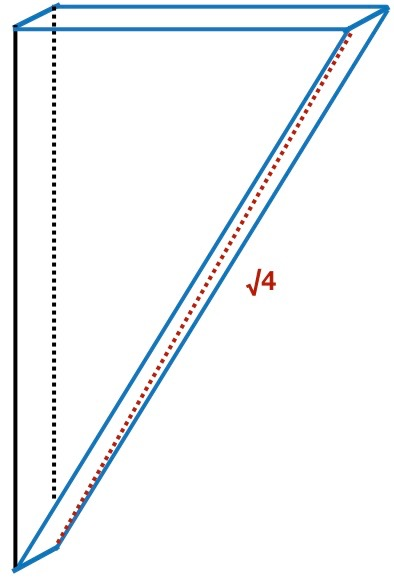We do know, however that the narrow vertical face is the shared surface between the two prisms, and we know that the area of its face must equal one square unit.  The length and width of this vertical face are obviously not equal.  Eliminating the 1 x 1 = 1 solution, we recognize that the length and width must be inverse values, like 2/1 x 1/2, or 3/1 x 1/3.  No other possibilities exist to give the product of 1.0000 when multiplied.

The length of the Vesica Piscis is √3, or 1.732, so the length of 2 is too long.  We eliminate 2 and all lengths greater than 2.

What numbers are inverse values that multiply to produce 1.000000 and whose larger value is more than 1 and less than 2?

Phi and phi, or 1.618033989 and .618033989, should come to mind.

Perhaps this unique pair numbers, which previously served as portals between linear and exponential dimension on our Nature-Based Number Line, will help us out again.  It would be convenient, if not coincidental, if they also serve as portals between the geometrical dimensions of Conscious Corporeality!Let us make the length of the shared surface between the two rectangles 1.618033989.  The inverse of 1.618033989 is .618033989, which would then be the width of the vertical face (seen above) and therefore, the depth of the prism itself (seen below).  Given these measurements, we can calculate the diagonal (red arrow below) to be √3.  Perfect!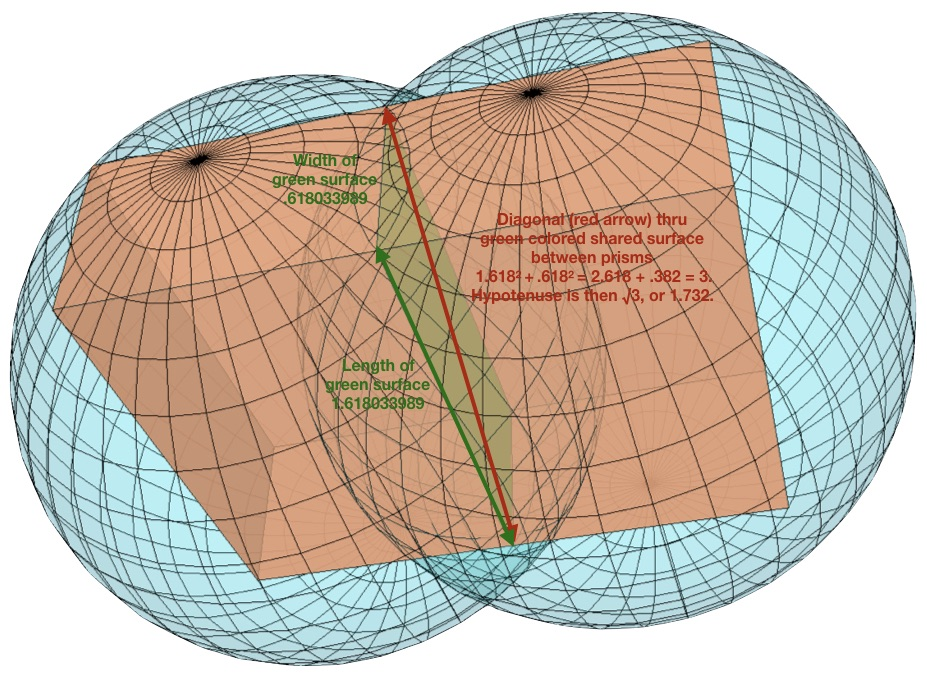If the width of the face of the prism’s inner slice is .618, and the hypotenuse through the prism is √4, we find its length by subtracting .618² from 4.

4 – .382 = 3.618.

Then we find the square root of 3.618, which is 1.9.  This makes 1.9 the hypotenuse of the large triangular face of the half-prism, seen outlined in magenta below.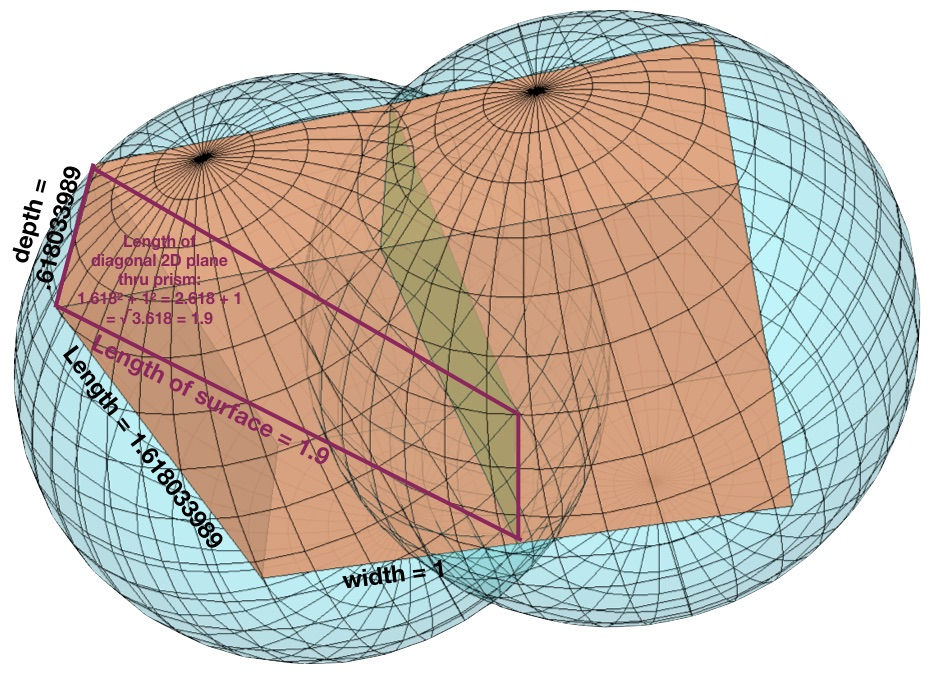To find the width of the half-prism, we repeat the process.  We take the length, 1.618 and square it to get 2.618 and subtract this from 3.618 (the square of 1.902).

3.618 – 2.618 = 1

We get 1, and the √1 is still 1.  The width of our prism is, therefore, 1 linear unit.

Diagonal plane (magenta) situated within the prism:
1.9² (length of surface) + .618² (depth of prism/width of surface) = 3.618 + .382 = 4.
Diagonal is √4, or 2 linear units.  The radius of each sphere is 1 linear unit, making the diameter of each sphere 2 linear units.  This fulfills our requirements.

Over all, the prism’s dimensions have been calculated to be 1.618 x 1 x .618.  Multiply all three dimensions to get one cubic unit.

1.618033990 x 1 x .618033989 = 1.000000Alright, we have a shared surface (2D) between the prisms measuring 1 square unit.

We have the volume (3D) of each of the two prisms’ measuring 1 cubic unit.

And we have the diagonal of the prisms measuring √4, or 2 linear units, equaling the diameter of the spheres into which they fit perfectly.  Also, the combined widths (left to right) of the two prisms = 2 or √4, same as the diameters of each circle.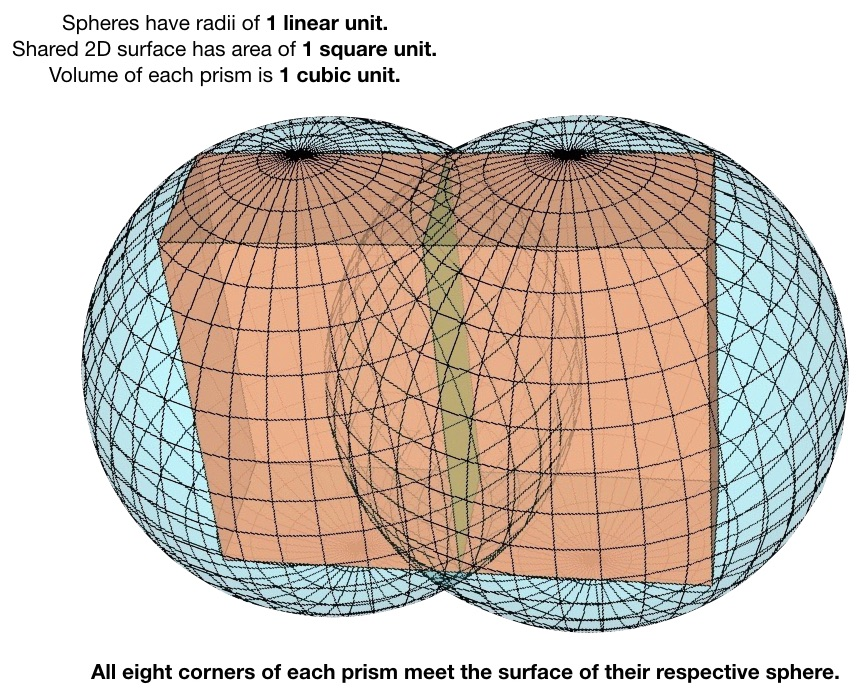All three dimensions of the unit 1 are accounted for.  In the process, we have done something else pretty remarkable.  We have related the golden ratio (Phi to 1 or 1 to phi) with pi, with the square root of the fourth dimension, representing Consciousness.

Phi is 1.6180339875…  and phi, its inverse, which also differs by 1, equals .6180339875…

Pi is the ratio of the circumference of a circle to its diameter, approximately 3.14159.

All three of these numbers, Phi, phi and pi are irrational numbers.The linear unit of one matches the 1D radii of the spheres with the 1D width of the prisms.

The 2D surface shared between the two prisms (measuring 1.618 x .618 = 1) fits precisely within the 2D circular disc where the bubbles meet (measuring √3, or ~1.732).

The 3D volumes relate (3D golden prisms connect at all of their eight points to the surface boundary of their respective 3D sphere).  From the 3D Pragmatic Schematic we discover the relationship between the values of pi, Phi and phi!

While the contiguous prisms symbolize content, the spheres symbolize the surrounding context.  To be Conscious of a thing, an event, an inspiration, etc., we must distinguish that which ‘counts’ from that which we are ‘discounting’.  For example, we may single out an object while ignoring its environment.  The 3D PS links content within context.

In Genesis, God “sees the light” and He “divides the light from the darkness.”  First there was darkness, and then there was light.  These opposites were compared and contrasted by God; though it could be any Observer/Creator.  For light to be seen, there must be a contrast between its past (darkness in left sphere) and current perception (light in the circular shared surface between the two spheres).  In retrospect, God names the light Day and the darkness Night; this happens in the right sphere.

Two contiguous instantiations are necessary for conscious apprehension. There is no light without dark, no hot without cold, no close without far, no up without down, no inside without outside, and no before without after, etc.  Perceptually contrasted incidents are illustrated in the 3D model as adjacent prisms within spheres.  The 3D model thus shows how time arises in the singling out of moments, one to the next, to the next, and so forth.

4D Consciousness comprehends this spectacular alignment of three units of one—linear, spatial, and volumetric.  Consciousness is metaphysical (beyond the 3 dimensions of the physical).  As Conscious observers, we transcend, while including, the three dimensions of unit one.

#### The Confluence of Geometry is Beautiful and Elegant

Leonard Euler is famous for his algebraic formula: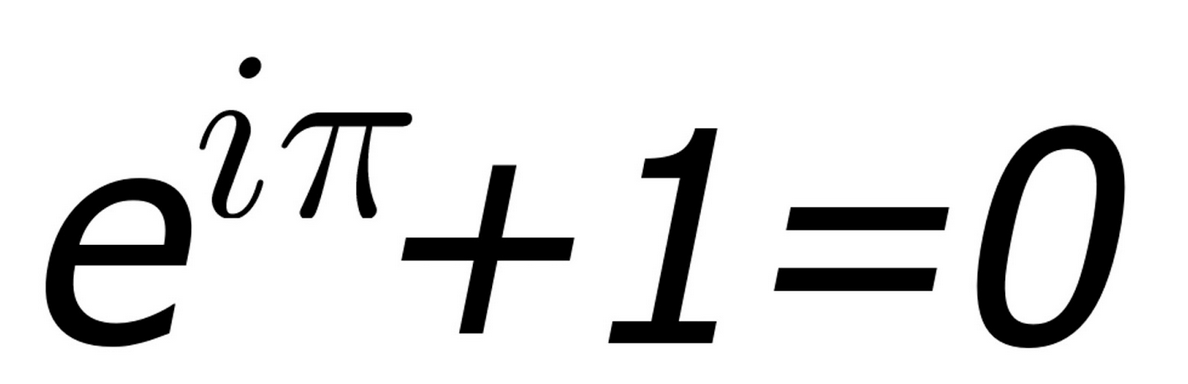This remarkable formula combines two transcendental numbers:

e, the base of natural logarithms, and

∏ or pi, which relates the radius of a circle or sphere to its circumference, surface area, and volume.

with i, the square root of -1, for which there is no solution; however, when squared i² = -1, a real number.

Euler ices the cake by including the integers 1 and 0!

What is achieved in this chapter is the geometrical correlation of the unit of 1 in three dimensions: 1D length, 2D area, and 3D volume.  Moreover, we related the Golden Ratio (1.618 is to 1 as 1 is to .618) with pi (which relates a circle’s radius to its circumference and area, and a sphere’s radius to its surface area and volume).

Recall that both Phi and pi are irrational numbers. The fact that their decimal extensions never repeat or end means we can never know their exact value (even as we truncate their values at three digits for convenience). Nevertheless, the imprecise numerical values of of Phi and pi are precisely connected geometrically. Because this elegant model spectacularly reveals the beautiful correlation of two otherwise seemingly unrelated irrational and almost mystical numbers, it offers significance in the field of geometry, just as Euler’s famous formula does in the field of algebra.

#### Symbology of the Geometry

Conscious minds perceive and make meaning of discrete moments as they fleetingly pass by.  The two contiguous prisms represent two sequential moments, which are compared and contrasted by one’s conscious mind..

Embodiment involves the overlap of birthing and dying.  Millions of cells in your body have died and millions more have been born, just while you read the two paragraphs of this chapter’s short section. The overlapped spheres represent these diametrically opposed, but complementary, processes.

The 3D model, which you can play with below, relates the Female/round/embodiment/OUTformation and the Male/angular/consciousness/INformation, demonstrating graphically the inextricable coordination, cooperation, and complementarity of the Female and Male.

#### Exploring the 3D Model for Yourself

A dream I had in 2004 showed me the 3D model and taught me most of the stuff written in this chapter.

Fifteen years later I met the amazing, young, genius, graphic artist, Abyssal Dionysus, at the grand opening of the Walter Russell Museum in Waynesbororo, VA.  While having lunch together, I saw some of his work and knew he was the one who could, at long last, create my 3D model.  I literally drew out what I wanted on the back of a napkin, and he got to work on it within days.

His contact info is provided with my highest recommendations.  Please check out his Facebook page and be prepared to be awed!!!

One last view of the vertical measurements before you explore the interactive model: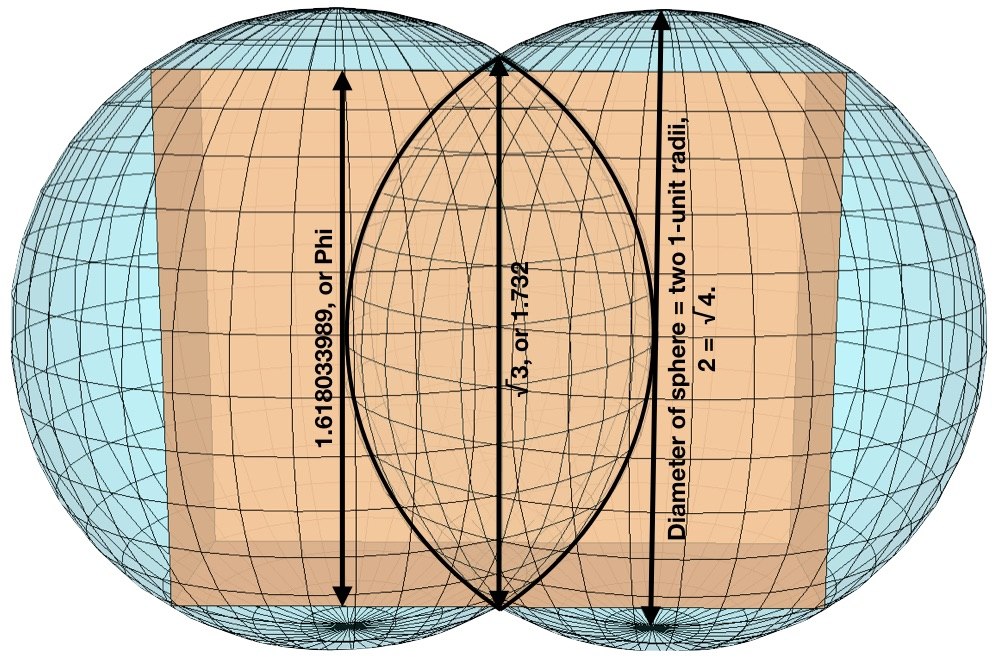Notice why the height of the prisms (1.618) needs to be a little shorter than the √3 (~1.732) measurement of the mandorla.  Hint: It has to do with the depth of the prism (.618).  The dimensions are precise and absolutely necessary for the pair of contiguous golden ratio prisms to connect Consciousness (Male prism with 90º angles) to Corporeality (Female spherical volumes) within a 3D version of a Sacred Geometry symbol.

Below is an interactive model.  It looks different from the screenshots seen above.  Unfortunately, the model as it appears above is incompatible with WordPress so a more rudimentary version has been supplied by the graphic designer for you to enjoy playing with.  Use your mouse to drag the image around to appreciate the model from all angles.